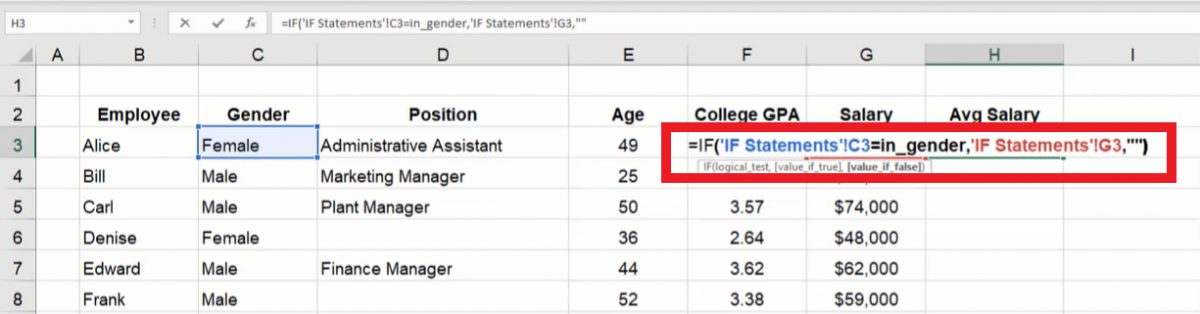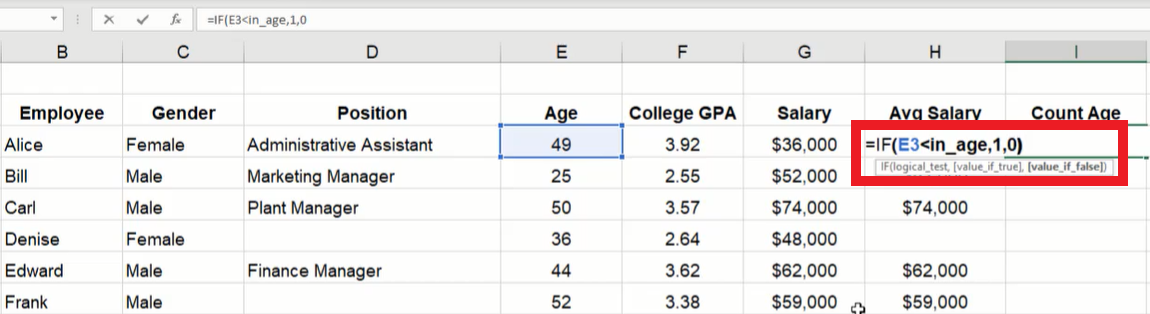# IF Statements

In this chapter, we’re going to look at conditional logic in Excel—specifically IF statements. We’ll start with a spreadsheet that lists employees for a company and includes their gender, position, age, college GPA, and current salary.

Use this workbook for the chapter.

## Dashboard Setup

Next, we’ll create a dashboard. The dashboard will include input and output cells. The input cells labeled as “Conditional Inputs” will include gender, age, retirement age, years window, bonus percent, raise over percent, raise under percent, and two GPA cells. The output cells labeled as “Conditional Outputs” will include average salary, number of employees, and employees eligible to retire. Finally, we’ll insert data into the input cells. (See Figure 17.1)

• Gender will contain a data list with Male and Female options created with the Data Validation tool.
• Age is 40.
• Retirement age is 65.
• Years window is 35.
• GPA related to bonus is 3.5.
• Bonus percent is 10%.
• GPA related to raise is 3.0.
• Raise percent over is 8%.
• Raise percent under is 3%.

Note: We can name these cells as we learned in the “Named Ranges” chapter. The cell names can be used hereafter in the formulas we will work on within this chapter.

## IF Data and Average

On the data spreadsheet shown in Figure 17.1, if we want to find the average salary of employees of a specific gender, we can use an IF function to list salaries in an empty column based on the dashboard’s input cell for employees’ gender. The IF function will check if an operation (equal to, less than, or greater than) involving two data values is true or false, and it can display a specific result if true or a different result if false. In this example, the logic test will check if the employee’s gender matches the gender displayed on the dashboard, and if true, it will display the employee’s salary. Then, we can reference the column’s cells in an AVERAGE function to find the total average salary for that gender.

1. Type =IF and a left parenthesis ( ( ) in an empty cell.
2. Select the employee’s gender cell for the logic test’s first value (C3 in Figure 17.3).
3. Type an equals sign ( = ).
1. In other situations, a less than symbol ( < ) or greater than symbol ( > ) may also be used to test values in an IF logic test.
4. Select the dashboard’s gender input cell or type the cell’s name for the logic test’s second value (the dashboard’s gender input cell in Figure 17.3).
1. The cell being compared in Figure 17.3 is from the dashboard sheet and has been named “in_gender.” If you named the cell differently, type the correct name. Otherwise, select the appropriate cell from the dashboard sheet.
5. Type a comma ( , ) and select the appropriate salary cell to display its value if the logic test is true (G3 in Figure 17.3).
6. Type a comma ( , ) and two double quotation marks ( " ).
1. The double quotation marks signify a text string. However, since no text is inserted between the marks, the cell will not display anything if the logic test is false.Figure 17.3

7. Type a right parenthesis ( ) ) and press Enter to complete the formula (=IF(C3=in_gender,G3,"")).
1. Notice the formula in Figure 17.3 displays additional text for the referenced cells. Excel requires the appropriate spreadsheet’s name to be included in the formula when referencing cells on another spreadsheet. In this case, the first referenced cell is 'IF Statements'!C3 (including the single quotation marks enclosing the spreadsheet name and followed by an exclamation mark).

Copy the formula down the column for each employee. Finally, create an AVERAGE function on the dashboard's Average salary output cell to reference this column's cells. The cell will display an overall average based on the gender selected in the dashboard's Gender input cell.

Note: There is a specific function known as AverageIFS that can calculate the same result using similar formula concepts in a single cell. Please research the AverageIFS function and learn more about how it can calculate the same result.

## IF Data and Sum

If we want to find the total employees under a specific age, we can use an IF function in an empty column to check if the employee’s age is less than the age specified on the dashboard’s Age input cell.

1. Type =IF and a left parenthesis ( ( ) in an empty cell.
2. Select the employee’s age cell for the logic test’s first value (E3 in Figure 17.4).Figure 17.4

3. Type a less than sign ( < ).
4. Select the dashboard’s age input cell or type the cell’s name for the logic test’s second value.
1. In Figure 17.4’s example, the referenced cell’s name is in_age.
5. Type a comma ( , ) and a 1.
6. Type a comma ( , ) and a 0.
7. Type a right parenthesis ( ) ) and press Enter to complete the formula (=IF(E3<in_age,1,0)).

Copy the formula down the column for each employee. Finally, create a SUM function on the dashboard's # of employees under the output cell to reference this column's cells. The cell will display a total of employees under the age specified in the Age input cell.

## IF Data and Operations

If we want to determine an employee’s raise based on their college GPA, we can write a formula to check the GPA and whether the raise will be 3% or 8%.

1. Type =IF and a left parenthesis ( ( ) in an empty cell.
2. Select the cell for the employee’s GPA (F3 in Figure 17.5).
3. Type a greater than sign ( > ).
4. Select the cell for GPA raise on the dashboard sheet.
1. In Figure 17.5, the referenced cell’s name is in_raise_gpa. If you named the cell differently, type the correct name. Otherwise, select the appropriate cell from the dashboard sheet.
5. Type a comma ( , ) and select the cell for raise percent over to indicate the raise percent for a GPA over the specified value.
1. In Figure 17.5, the referenced cell’s name is in_raise_over.
6. Type a comma ( , ) and select the cell for raise percent under to indicate the raise percent for a GPA under the specified value.
1. In Figure 17.5, the referenced cell’s name is in_raise_under.
7. Type a right parenthesis ( ) ) to complete the formula (=IF(F3>in_raise_gpa,in_raise_over,in_raise_under)).

Format the cell as a percentage and copy the formula down the column for each employee.

If we want to find the raise dollar amount, we can multiply the percentage by the current salary in a new cell. Alternatively, we can copy or add to the raise percentage formula to multiply by the salary (=IF(F3>in_raise_gpa,in_raise_over,in_raise_under)*G3).

If we want to know the total new salary, we can modify the formula to add the IF calculation to 1 which signifies 103% or 108% (depending on the percent returned based on the employee’s GPA). The formula will calculate the total new salary in a single cell.

1. Type an equals symbol ( = ) and a left parenthesis ( ( ) in a new cell.
2. Type 1 to represent 100% and an addition sign ( + ).
3. Type IF and a second left parenthesis ( ( ).
4. Select the cell for the employee’s GPA (F3 in Figure 17.6).
5. Type a greater than sign ( > ).
6. Select the cell for GPA raise on the dashboard sheet.
1. In Figure 17.6, the referenced cell’s name is in_raise_gpa. If you named the cell differently, type the correct name. Otherwise, select the appropriate cell from the dashboard sheet.
7. Type a comma ( , ) and select the cell for raise percent over to indicate the raise percent for a GPA over the specified value.
1. In Figure 17.6, the referenced cell’s name is in_raise_over.
8. Type a comma ( , ) and select the cell for raise percent under to indicate the raise percent for a GPA under the specified value.
1. In Figure 17.6, the referenced cell’s name is in_raise_under.
9. Type a right parenthesis ( ) ) to close the IF logic formula.
10. Type a second right parenthesis ( ) ) to close the addition operation.
11. Type an asterisk ( * ) for multiplication.
12. Select the cell with the employee’s current salary (G3 in Figure 17.6).
13. Press Enter to complete the formula (=(1+IF(F3>in_raise_gpa,in_raise_over,in_raise_under))*G3).